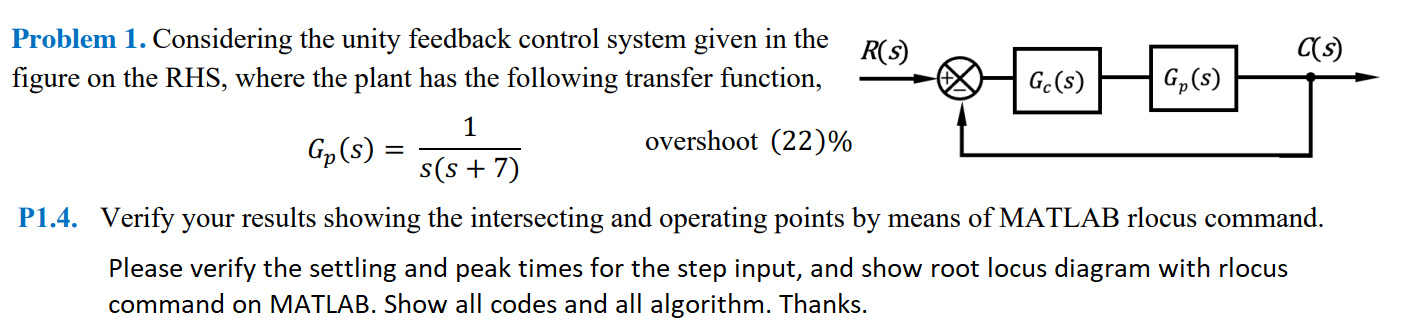# (Solved): Gc(s)=K Problem 1. Considering the unity feedback control system given in the ...???????

Gc(s)=K
Problem 1. Considering the unity feedback control system given in the figure on the RHS, where the plant has the following transfer function, $G_{p}(s)=\frac{1}{s(s+7)} \quad \text { overshoot }(22) \%$ P1.4. Verify your results showing the intersecting and operating points by means of MATLAB rlocus command. Please verify the settling and peak times for the step input, and show root locus diagram with rlocus command on MATLAB. Show all codes and all algorithm. Thanks.

We have an Answer from Expert